# PSEB 8th Class Maths Solutions Chapter 11 Mensuration Ex 11.2

Punjab State Board PSEB 8th Class Maths Book Solutions Chapter 11 Mensuration Ex 11.2 Textbook Exercise Questions and Answers.

## PSEB Solutions for Class 8 Maths Chapter 11 Mensuration Ex 11.2

1. The shape of the top surface of a table is a trapezium. Find its area if its parallel sides are 1 m and 1.2 m and perpendicular distance between them is 0.8 m.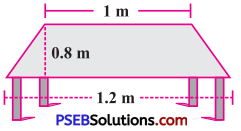Solution:
Area of top surface of a table = $$\frac {1}{2}$$ × (sum of parallel sides) × perpendicular distance between the parallel sides
= $$\frac {1}{2}$$ × (1.2 + 1) × 0.8
= $$\frac {1}{2}$$ × 2.2 × 0.8
= 0.88 m2
Hence, area of top surface of table is 0.88 m2.2. The area of a trapezium is 34 cm2 and the length of one of the parallel sides is 10 cm and its height is 4 cm. Find the length of the other parallel side.
Solution: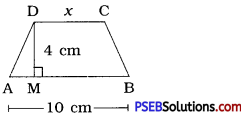Let the length of the other parallel side be xcm.
Area of trapezium
= $$\frac {1}{2}$$ × (sum of parallel sides) × height
= $$\frac {1}{2}$$ × (10 + x) × 4
= (10 + x) × 2
= 20 + 2x
Area of trapezium = 34 cm2 (given)
∴ 20 + 2x = 34
∴ 2x = 34 – 20
∴ 2x = 14
∴ x = 7
Hence, length of the other parallel side is 7 cm.

3. Length of the fence of a trapezium-shaped field ABCD is 120 m. If BC = 48 m, CD = 17 m and AD = 40 m, find the area of this field. Side AB is perpendicular to the parallel sides AD and BC.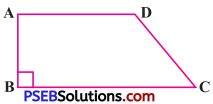Solution:
Perimeter of a field = length of fence of a held
∴ AB + BC + CD + DA = 120
∴ AB + 48 + 17 + 40 = 120
∴ AB + 105 = 120
∴ AB = 120 – 105
∴ AB = 15 m
Now, Area of trapezium ABCD = $$\frac {1}{2}$$ × (sum of parallel sides)
× perpendicular distance between the parallel sides
= $$\frac {1}{2}$$ × (AD + BC) × AB
= $$\frac {1}{2}$$ × (40 + 48) × 15
= $$\frac {1}{2}$$ × 88 × 15 = 660 m2
Hence, area of the field is 660 m2.4. The diagonal of a quadrilateral shaped field is 24 m and B the perpendiculars dropped on it from c the remaining opposite vertices are 8 m and 13 m. Find the area of the field.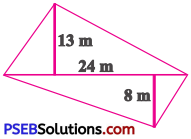Solution:
Area of Δ ABD + Area of Δ BCD
= $$\frac {1}{2}$$ × BD × AM + $$\frac {1}{2}$$ × BD x CN
= $$\frac {1}{2}$$ × 24 × 13 + $$\frac {1}{2}$$ × 24 × 8
= 12 × 13 + 12 × 8
= 156 + 96
= 252 m2
Hence, area of the field is 252 m2.

5. The diagonals of a rhombus are 7.5 cm and 12 cm. Find its area.
Solution:
d1 = 7.5 cm, d2 = 12 cm
Area of a rhombus = $$\frac {1}{2}$$ × d1 × d2
= $$\frac {1}{2}$$ × 7.5 × 12
= 7.5 × 6 = 45 cm2.
Hence, area of rhombus is 45 cm2.6. Find the area of a rhombus whose side is 5 cm and whose altitude is 4.8 cm. If one of its diagonals is 8 cm long, find the length of the other diagonal.
Solution:
Rhombus is a parallelogram too.
∴ Area of rhombus = base × height
= 5 × 4.8
= 5 × $$\frac {48}{10}$$
= 24 cm2
Now, area of rhombus = $$\frac {1}{2}$$ × d1 × d2
∴ 24 = $$\frac {1}{2}$$ × 8 × d2
∴ 24 = 4 × d2
∴ d2 = $$\frac {24}{4}$$ = 6 Cm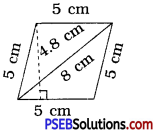Hence, length of the other diagonal of rhombus is 6 cm.

7. The floor of a building consists of 3000 tiles which are rhombus-shaped and each of its diagonals are 45 cm and 30 cm in length. Find the total cost of polishing the floor, if the cost per m2 is ₹4.
Solution:
The shape of a floor tile is rhombus.
d1 = 45 cm, d2 = 30 cm
Area of a tile = $$\frac {1}{2}$$ × d1 × d2
= $$\frac {1}{2}$$ × 45 × 30
= 45 × 15
= 675 cm2
Now, floor of a building consists of total 3000 tiles.
∴ Area of floor = Number of tiles × Area of one tile
= 3000 × 675
= 20,25,000 cm2
1cm = $$\frac {1}{100}$$m
∴ 1cm2 = $$\frac{1}{100 \times 100}$$m2 = $$\frac {1}{10000}$$m2
∴ 20,25,000 cm2 = $$\frac{2025000}{10000}$$m2
= 202.5 m2
∴ Cost of polishing the floor = ₹ 4 per m2
∴ Total cost of polishing the floor
= ₹ 4 × 202.5 = ₹ 810
Hence, total cost of polishing the floor is ₹ 8108. Mohan wants to buy a trapezium-shaped field. Its side along the river is parallel to and twice the sidealong the road. If the area of this field is 10,500 m2 and the perpendicular distance between the two parallel sides is 100 m, And the length of the side along the river.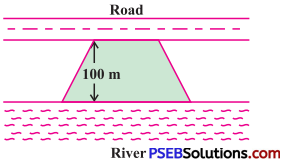Solution:
The side along the river is parallel to and twice the side along the road.
Let the length of side along the road be x m.
Then, the length of side along the river = 2xm
Area of trapezium
= $$\frac {1}{2}$$ × (sum of parallel sides) × height
= $$\frac {1}{2}$$ × (x + 2x) × 100
= $$\frac {1}{2}$$ × 3x × 100
= 3x × 50 = 150 x
But, area of field = 10500 m2 (given)
∴ 150x = 10500
∴ x = $$\frac {10500}{150}$$
∴ x = 70m
∴ 2x = 2 × 70 = 140 m
Hence, the length of the side along the river is 140 m.

9. Top surface of a raised platform is in the shape of a regular octagon as shown in the figure. Find the area of the octagonal surface.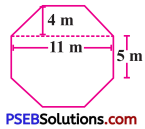Solution:
Let us, divide regular octagon into two congruent trapezium and a rectangle. Then add areas of all these three shapes.
Here, height of trapezium = 4 m Length of two parallel sides = 11 m and 5 m respectively.
∴ Area of a trapezium = $$\frac {1}{2}$$ × (11 + 5) × 4
= $$\frac {1}{2}$$ × 16 × 4
= 8 × 4
= 32 m2
∴ Area of two trapeziums = 2 × 32
= 64m2 …….. (i)
Area of a rectangle = length × breadth
= 11 × 5 = 55m2 …….. (ii)
∴ Area of regular octagonal platform
= (64 + 55) m2 [From (i) and (ii)]
= 119 m210. There is a pentagonal-shaped park as shown in the figure. For finding its area Jyoti and Kavita divided it in two different ways.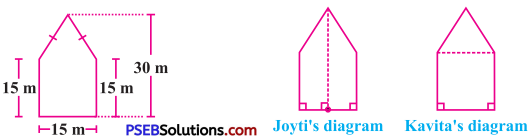Find the area of this park using both ways. Can you suggest some other way of finding its area?
Solution:
Jyoti has divided given pentagon into two congruent trapeziums.
∴ Area of a trapezium = $$\frac {1}{2}$$ × (sum of parallel sides) × perpendicular distance between two parallel sides
= $$\frac {1}{2}$$ × (15 + 30) × $$\frac {15}{2}$$
= $$\frac {1}{2}$$ × 45 × $$\frac {15}{2}$$
= $$\frac {675}{4}$$ m2
∴ Area of two trapeziums = 2 × $$\frac {675}{4}$$
= $$\frac {675}{2}$$
= 337.5 m2
Now, Kavita has divided given pentagon into one triangle and the other square.
∴ Area of a triangle = $$\frac {1}{2}$$ × b × h
= $$\frac {1}{2}$$ × 15 × 15
= $$\frac {225}{2}$$
= 112.5m2 …………….(i)
∴ Area of a square = (side)2
= (15)2 = 15 × 15
= 225 m2 ……….(ii)
Area of a pentagon
= (112.5 + 225) m2 [From (i) and (ii)]
= 337.5 m2
Now, let us use our idea and find another method to find area of a given pentagon. Here, we have divided given pentagon into three triangles.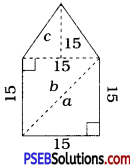Area of ∆ a = $$\frac {1}{2}$$ × 15 × 15
= $$\frac {225}{2}$$
= 112.5m2 … (i)
Area of ∆ b = $$\frac {1}{2}$$ × 15 × 15
= $$\frac {225}{2}$$
= 112.5 m2 ………… (ii)
Area of ∆ c = $$\frac {1}{2}$$ × 15 × 15
= $$\frac {225}{2}$$
= 112.5 m2 ……. (iii)
From (i), (ii) and (iii) the area of a given pentagon
= (i) + (ii) + (iii)
= (112.5 + 112.5 + 112.5) m2
= 337.5 m2
Hence, area of the given pentagon is 337.5 m2.
[Note : By using such ideas, i.e., dividing any shape into convienient shapes, you can find area of given figure.]11. Diagram of the adjacent picture frame has outer dimensions = 24 cm × 28 cm and inner dimensions 16 cm × 20 cm. Find the area of each section of the frame, if the width of each section is same.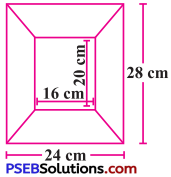Solution:
Here, picture frame is divided into four trapeziums, such that a, b, c and d.
The sides of opposite trapeziums have same measurement so they have equal area.
∴ Area of a = area of c and area of b = area of d

For trapezium a and c:
Length of parallel sides = 24 cm, 16 cm
Height = $$\frac{28-20}{2}=\frac{8}{2}$$ = 4 cm
∴ Area of trapezium a
= $$\frac {1}{2}$$ × (sum of parallel sides) × perpendicular distance between parallel sides
= $$\frac {1}{2}$$ × (24 + 16) × 4
= $$\frac {1}{2}$$ × 40 × 4
= 80 cm2
So area of trapezium c = 80 cm2.

For trapezium b and d:
Length of parallel sides = 28 cm, 20 cm
Height = $$\frac{24-16}{2}=\frac{8}{2}$$ = 4 cm
∴ Area of trapezium b
= $$\frac {1}{2}$$ × (sum of parallel sides) × perpendicular distance between parallel sides
= $$\frac {1}{2}$$ × (28 + 20) × 4
= $$\frac {1}{2}$$ × 48 × 4
= 96 cm2
So area of trapezium d = 96 cm2.
Hence, area of each section of frame is as follows.
Area of section a = 80 cm2
Area of section b = 96 cm2
Area of section c = 80 cm2
Area of section d = 96 cm2
To find the total surface area, we find the area of each face and then add them.